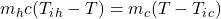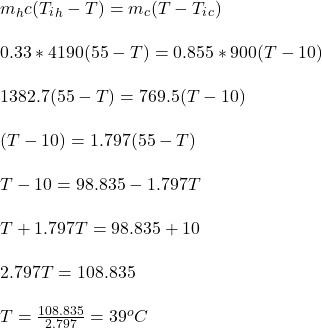A person pours 330 g of water at 55°C into an 855-g aluminum container with an initial temperature of 10°C. The specific heat of aluminum is

Question

A person pours 330 g of water at 55°C into an 855-g aluminum container with an initial temperature of 10°C. The specific heat of aluminum is 900 J/(kg・ΕK) and that of water is 4190 J/(kg・ΕK). What is the final temperature of the system, assuming no heat is exchanged with the surroundings?

in progress 0
5 months 2021-08-09T15:42:52+00:00 1 Answers 39 views 0

The final temperature of the system is 39⁰C

Explanation:

Applying principle of conservation of heat energy;

Heat loss by a hot body = heat gained by a cold bodywhere;

Mh is the mass of the hot fluid = 330 g = 0.33 kg

Mc  is the mass of the cold fluid = 855-g = 0.855 kg

Tih is the initial temperature of the hot fluid = 55°C

Tic is the initial temperature of the cold fluid = 10°C

T is the final temperature of the mixture

Substitute the given values and solve for TTherefore, the final temperature of the system is 39⁰C